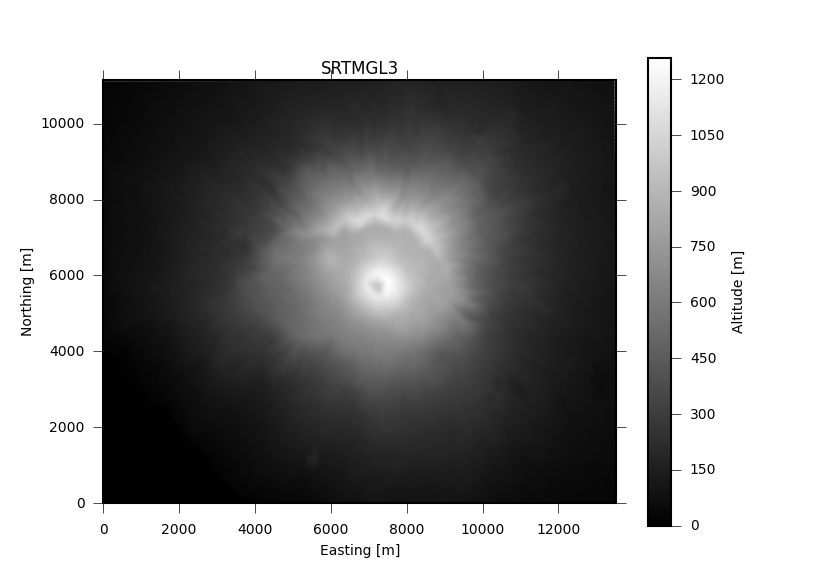# Geographical datasets¶

## Topography¶

The `pyrocko.dataset.topo` subpackage offers quick access to some popular global topography datasets.

The following example draws gridded topography for Mount Vesuvius from SRTMGL3 (, , , ) in local cartesian coordinates.

```import numpy as num
from pyrocko import plot, orthodrome as od
from pyrocko.dataset import topo

lon_min, lon_max, lat_min, lat_max = 14.34, 14.50, 40.77, 40.87
dem_name = 'SRTMGL3'

tile = topo.get(dem_name, (lon_min, lon_max, lat_min, lat_max))

# geographic to local cartesian coordinates
lons = tile.x()
lats = tile.y()
lons2 = num.tile(lons, lats.size)
lats2 = num.repeat(lats, lons.size)
norths, easts = od.latlon_to_ne_numpy(lats, lons, lats2, lons2)
norths = norths.reshape((lats.size, lons.size))
easts = easts.reshape((lats.size, lons.size))

# plot it
plt = plot.mpl_init(fontsize=10.)
fig = plt.figure(figsize=plot.mpl_papersize('a5', 'landscape'))
axes = fig.add_subplot(1, 1, 1, aspect=1.0)
cbar = axes.pcolormesh(easts, norths, tile.data,
fig.colorbar(cbar).set_label('Altitude [m]')
axes.set_title(dem_name)
axes.set_xlim(easts.min(), easts.max())
axes.set_ylim(norths.min(), norths.max())
axes.set_xlabel('Easting [m]')
axes.set_ylabel('Northing [m]')
fig.savefig('topo_example.png')
```Footnotes

  Farr, T. G., and M. Kobrick, 2000, Shuttle Radar Topography Mission produces a wealth of data. Eos Trans. AGU, 81:583-585.
  Farr, T. G. et al., 2007, The Shuttle Radar Topography Mission, Rev. Geophys., 45, RG2004, doi:10.1029/2005RG000183. (Also available online at http://www2.jpl.nasa.gov/srtm/SRTM_paper.pdf)
  Kobrick, M., 2006, On the toes of giants-How SRTM was born, Photogramm. Eng. Remote Sens., 72:206-210.
  Rosen, P. A. et al., 2000, Synthetic aperture radar interferometry, Proc. IEEE, 88:333-382.

## GSHHG coastal database¶

The GSHHG database is a high-resolution geography data set. We implement functions to extract coordinates of landmasks.

Classes covered in this example:
```import numpy as num
from pyrocko.dataset.gshhg import GSHHG
from matplotlib import pyplot as plt

gshhg = GSHHG.intermediate()
# gshhg = GSHHG.full()
# gshhg = GSHHG.low()

gshhg.is_point_on_land(lat=32.1, lon=44.2)

# Create a landmask for a regular grid
lats = num.linspace(30., 50., 500)
lons = num.linspace(2., 10., 500)

lon_grid, lat_grid = num.meshgrid(lons, lats)
coordinates = num.array([lat_grid.ravel(), lon_grid.ravel()]).T

plt.show()
```

Download `gshhg_example.py`

Footnotes

  Wessel, P., and W. H. F. Smith, A Global Self-consistent, Hierarchical, High-resolution Shoreline Database, J. Geophys. Res., 101, #B4, pp. 8741-8743, 1996.

## Tectonic plates and boundaries (PB2003)¶

An updated digital model of plate boundaries  offers a global set of present plate boundaries on the Earth. This database used in `pyrocko.automap`.

Classes covered in this example:
```from pyrocko.dataset.tectonics import PeterBird2003

poi_lat = 12.4
poi_lon = 133.5

PB = PeterBird2003()
plates = PB.get_plates()

for plate in plates:
if plate.contains_point((poi_lat, poi_lon)):
print(plate.name)
print(PB.full_name(plate.name))

'''
>>> PS
>>> Philippine Sea Plate
'''
```

Download `tectonics_example.py`

Footnotes

  Bird, Peter. “An updated digital model of plate boundaries.” Geochemistry, Geophysics, Geosystems 4.3 (2003).

## Global strain rate (GSRM1)¶

Access to the global strain rate data set from Kreemer et al. (2003) .

Classes to be covered in this example:

Warning

To be documented by example!

Footnotes

  Kreemer, C., W.E. Holt, and A.J. Haines, “An integrated global model of present-day plate motions and plate boundary deformation”, Geophys. J. Int., 154, 8-34, 2003.